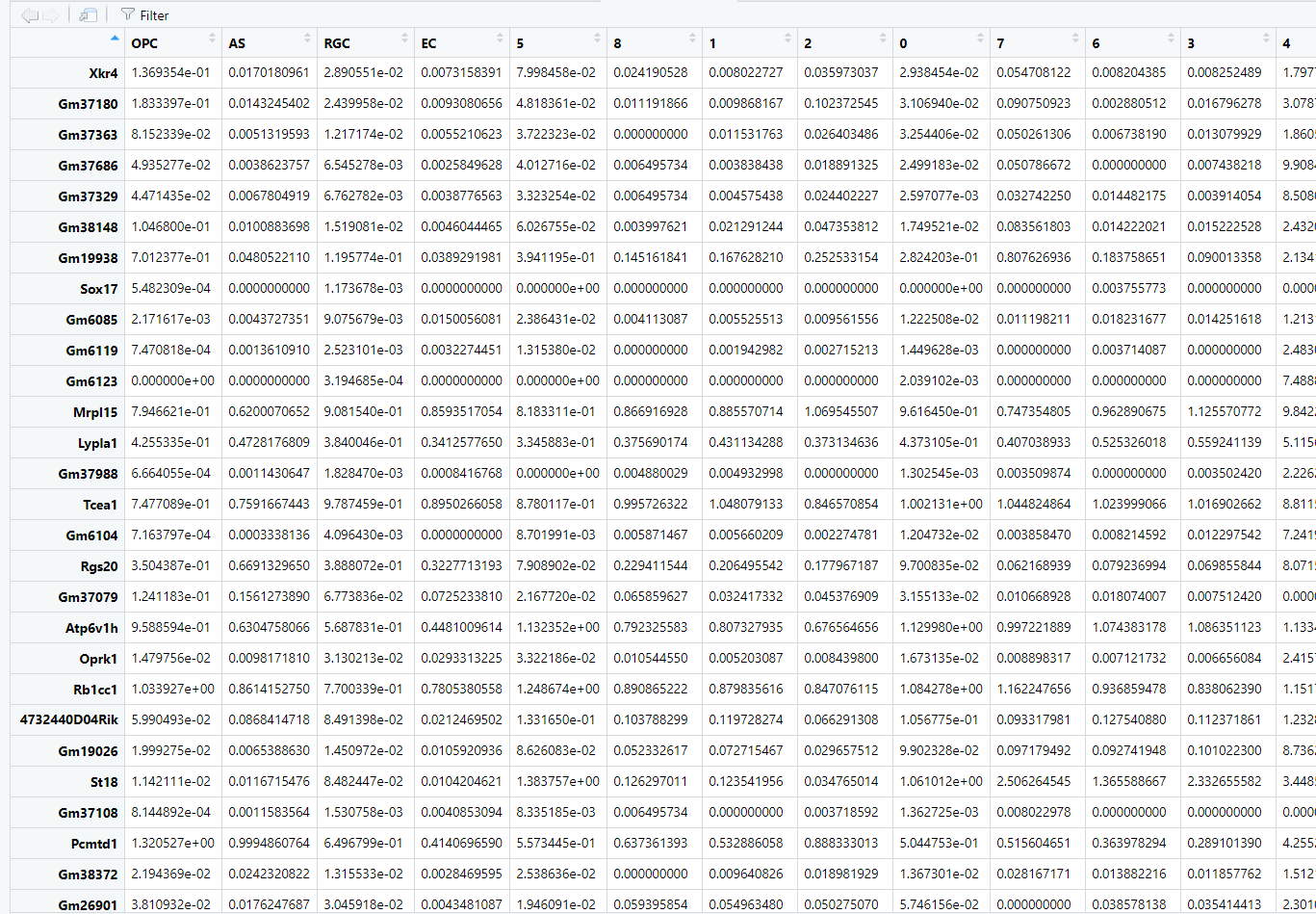# Which correlation method to compute the correlation score between different clusters of Sc-RNAseq data?

I'm analyzing single cell rna-seq data and trying to compute the correlation score between different clusters. Wondering how to choose the correlation method("pearson" (default), "kendall", or "spearman") of the Cor function?

I'm a new comer in bioinformatics and know little about these arithmetics. Can anyone give me some advice or show me the code to decide which method to choose for my data matrix?

Below is my gene expression matrix: rows are gene names,and columns are clusters.I use the default Peasrson method to do analysis,but I really don't know if it is right.CorOb.cor.exp <- as.data.frame(cor(CorOb.av.exp))
CorOb.cor.exp$$x <- rownames(CorOb.cor.exp) neupeptide.cor.df <- tidyr::gather(data = CorOb.cor.exp, y, correlation,CorOb.cor.exp$$x)
a <- ggplot(neupeptide.cor.df, aes(x, y, fill = correlation)) + geom_raster()+scale_fill_gradientn(colors = c("blue", "white", "red"))+
theme(axis.text.x = element_text(angle = 45, vjust = 1,
size = 12, hjust = 1))+
coord_fixed()


a

• Cross-posted: github.com/satijalab/seurat/issues/2198
– haci
Oct 14, 2019 at 11:41
• I don't think github issues are the best place to ask for general help such as this. Oct 17, 2019 at 15:47

Disclaimer: This is not a complete answer but more a comment, posting as an answer because of character restrictions for comments. Also, I am not a statistican but a molecular biologist who did quite some reading/courses on stats.

Two scRNA-seq tools, namely SingleR and RCA (Reference Component Analysis, Li et. al, 2017) compute correlations of bulk transcriptomic data to single cells; for this they use Spearman correlation and Pearson correlation respectively (The two tools use the same bulk transcriptome reference). I am using these two tools for different purposes so I did not really compare their results, however, single cell clusters that I compute using the reference components (calcuated with RCA) is in good agreement with single cells labels computed with RCA (adjusted rand index of 0.81).

In addition to that, please take a look at scMatch: a single-cell gene expression profile annotation tool using reference datasets, they claim (scMatch computes bulk - single cell correlations like SingleR and RCA):

From this comparison we found Spearman’s correlation coefficient outperformed Pearson’s correlation for all six libraries.

This question does not have a definite answer. Rather, the answer depends heavily on the assumptions you wish to make regarding your data.

Given a pair of random variables $$x$$ and $$y$$, Pearson's rho has a value of 1 when there is a linear relationship between $$x$$; i.e. $$y = x\beta + c$$, where $$c$$ is a constant, and there is no noise. Note that the slope, $$\beta$$, and intercept, $$c$$, of this relationship do not affect the correlation coefficient. With Spearman's rho, we first compute the rank of each element in $$x$$ and $$y$$. We then calculate the Pearson correlation between these ranks.

Kendall's tau correlation coefficient also deals with the ranks of $$x$$ and $$y$$, but is slightly more complex. The most relevant difference in this case is likely that tau is better in the case of ties, and may be more robust for low sample sizes. In most cases, Kendall's and Spearman's are likely to give very similar results

If you wish to assess only linear relationships between expression profiles, Pearson's is the most appropriate. If you wish to allow for non-linear effects, Spearman or Kendall is most appropriate (Kendall if the data contains ties).

Pearson correlation evaluates two continuous variables. Spearman correlation is often used to evaluate ordinal variables. Kendall correlation is a nonparametric test for two continuous variables.